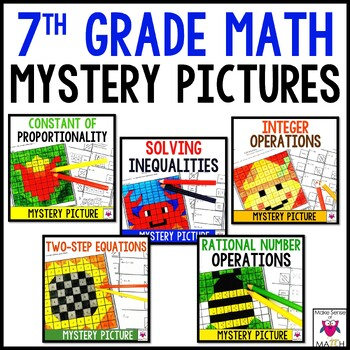# 7th Grade Math Coloring Activities | Mystery Pictures

Rated 4.92 out of 5, based on 12 reviews
12 Ratings;
7th, Homeschool
Subjects
Resource Type
Standards
Formats Included
• Zip
Pages
37 pages
\$9.50
List Price:
\$12.00
You Save:
\$2.50
Bundle
\$9.50
List Price:
\$12.00
You Save:
\$2.50
Bundle
Report this resource to TPT

#### Products in this Bundle (5)

##### Also included in
1. Looking to save time, money and sanity? Then this 7th grade math curriculum and activities bundle is for you! This curriculum is aligned to the 7th grade common core math standards. Each unit includes: guided notes, assessments/worksheets and activities such as: task cards, stations, mystery pictur
Price \$250.00Original Price \$403.00Save \$153.00

### Description

Looking for an engaging way to practice 7th grade math concepts? Students will love these 7th grade math activities as they practice integer operations, rational number operations, two step equations, solving inequalities and identifying the constant of proportionality. Students will color a grid according to their answer and reveal a mystery picture.

Includes All of This

Integer Operations

• 30 integers problems (add, subtract, multiply and divide)
• 1 coloring grid
• 30 number lines to accompany each question (optional use)

Rational Number Operations

• 20 rational numbers operations to simplify (positive and negative fractions)
• 1 coloring grid

Two Step Equations

• 20 two-step equations with integers
• 1 coloring grid
• Separate space to check their work (optional use)

Solving Inequalities

• 20 inequalities with integers (14 inequalities to solve and 6 graphs)
• 1 coloring grid
• Separate space to check their work (optional use)

Constant of Proportionality

• 20 constants of proportionality to identify
• 1 coloring grid

Highlights

• No prep
• Perfect for a substitute
• Extra credit
• Homework
• In-class work
• Engaging

FOLLOW ME for more great products to make sense of math!

Remember that leaving feedback earns you points toward FREE TPT purchases!

Please feel free to contact me with any questions!

Michelle,

Make Sense of Math

makesenseofmath@yahoo.com

Total Pages
37 pages
Included
Teaching Duration
N/A
Report this resource to TPT
Reported resources will be reviewed by our team. Report this resource to let us know if this resource violates TPT’s content guidelines.

### Standards

to see state-specific standards (only available in the US).
Understand subtraction of rational numbers as adding the additive inverse, 𝘱 – 𝘲 = 𝘱 + (–𝘲). Show that the distance between two rational numbers on the number line is the absolute value of their difference, and apply this principle in real-world contexts.
Apply properties of operations as strategies to add and subtract rational numbers.
Apply and extend previous understandings of multiplication and division and of fractions to multiply and divide rational numbers.
Understand that multiplication is extended from fractions to rational numbers by requiring that operations continue to satisfy the properties of operations, particularly the distributive property, leading to products such as (–1)(–1) = 1 and the rules for multiplying signed numbers. Interpret products of rational numbers by describing real-world contexts.
Understand that integers can be divided, provided that the divisor is not zero, and every quotient of integers (with non-zero divisor) is a rational number. If 𝘱 and 𝘲 are integers, then –(𝘱/𝘲) = (–𝘱)/𝘲 = 𝘱/(–𝘲). Interpret quotients of rational numbers by describing real-world contexts.